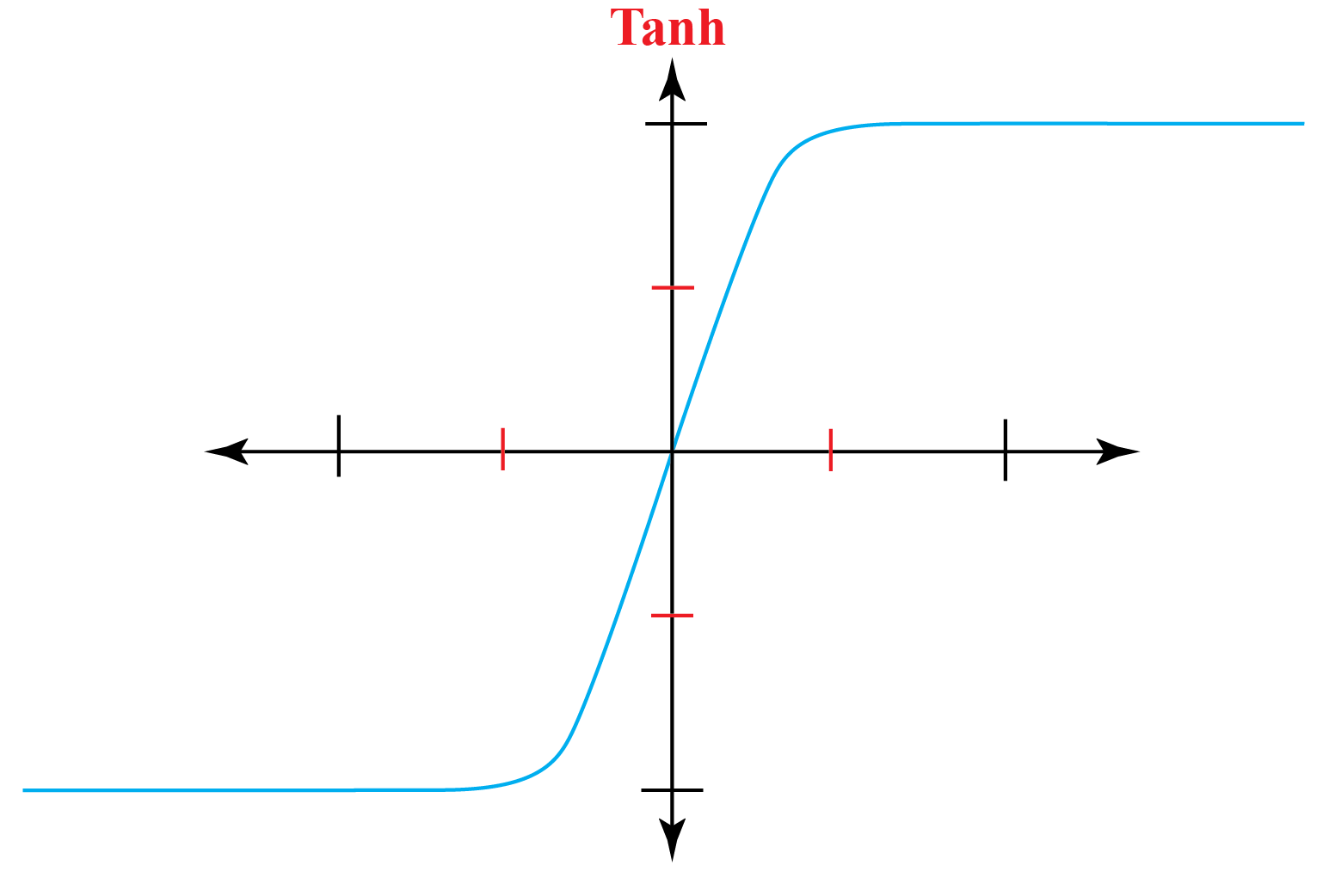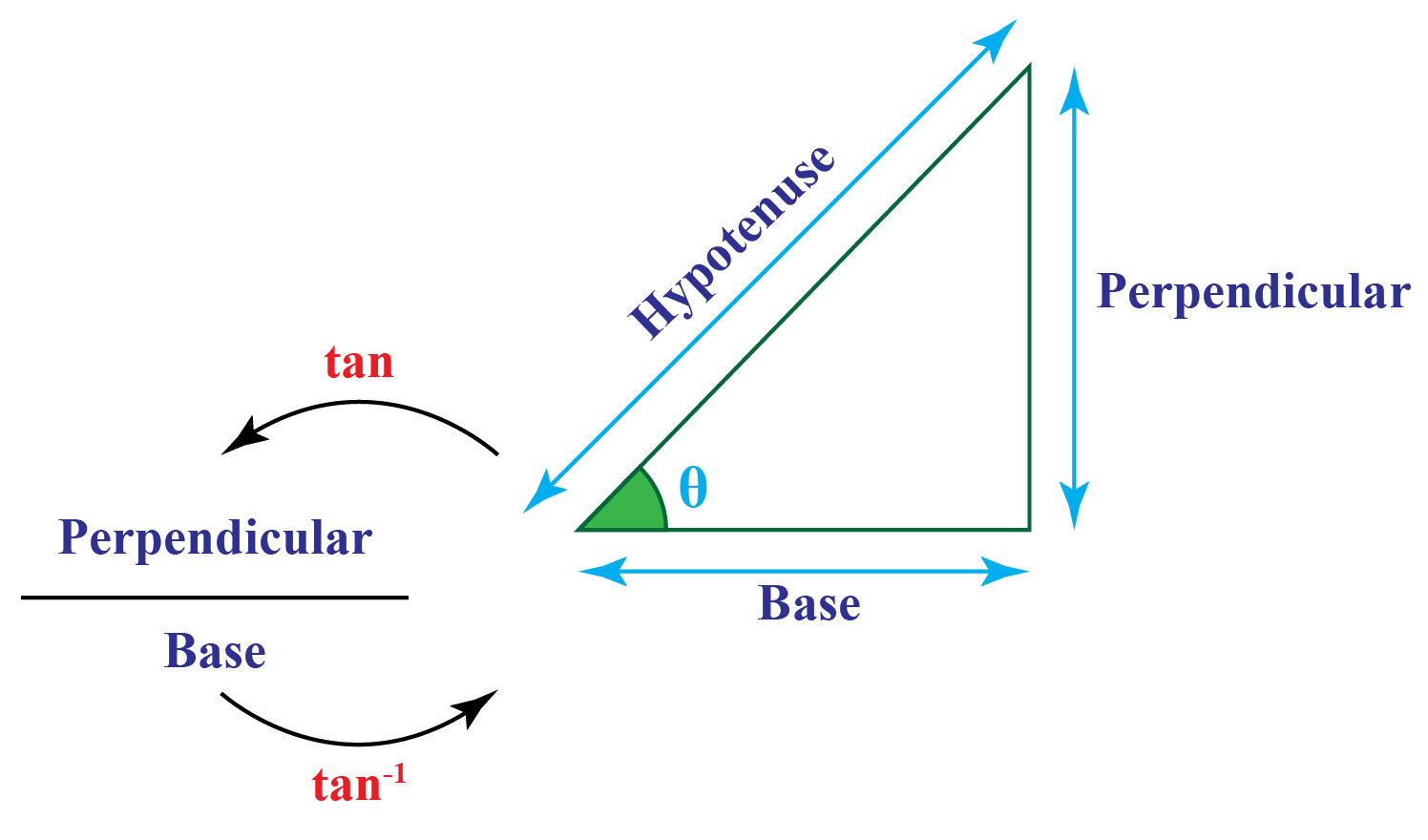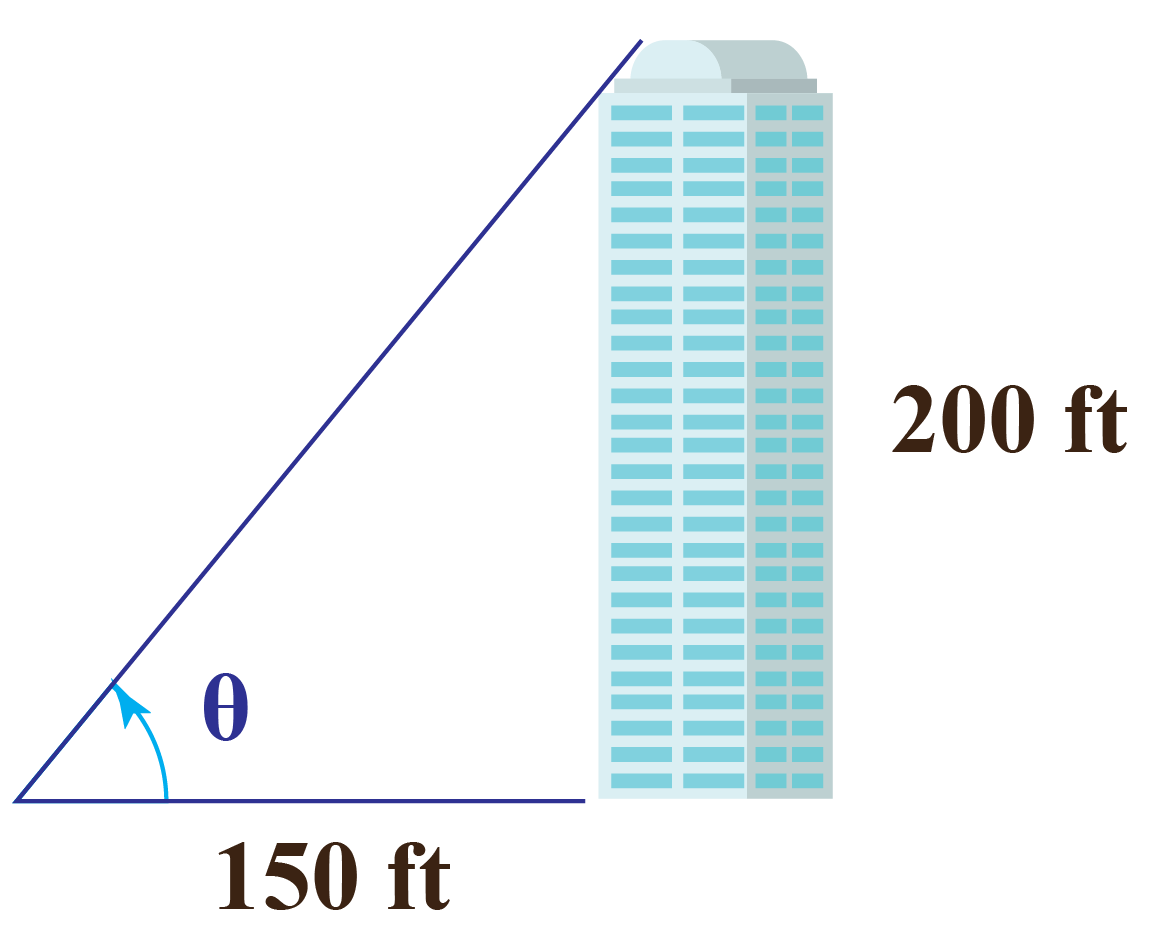# Tanh

Tanh

You have already learned about trigonometric functions and their properties. Unlike trigonometric functions, hyperbolic functions are defined for the hyperbola.

In this short lesson, you will learn about Tanh hyperbolic function and its properties including tanh function, tanh calculator, tanh formula, and tanh equation.

You can see the graphical representation of the Tanh function in the image below.## Lesson Plan

 1 What Is Meant by Tanh? 2 Important Notes 3 Solved Examples on Tanh Function 4 Thinking Out of the Box! 5 Interactive Questions on Tanh Function

## What Is Meant by Tanh?

Tanh is a hyperbolic function that is pronounced as "tansh."

The function Tanh is the ratio of Sinh and Cosh.

$\tanh=\dfrac{\sinh}{\cosh}$

We can even work out with exponential function to define this function.

$\tanh=\dfrac{e^{x}-e^{-x}}{e^{x}+e^{-x}}$

## What Are the Formulas for Tanh Function?

There is more than one formula for the Tanh function.

Here are a few of the Tanh formulas.

 $\tanh{(-x)}=-\tanh{(x)}$
 $\tanh{(x \pm y)}=\dfrac{\tanh{(x)} \pm \tanh{(y)}}{1 \pm \tanh{(x)}\tanh{(y)}}$
 $\tanh{(2x)}=\dfrac{2\tanh{(x)}}{1 + \tanh^{2}{(x)}}$
 $\tanh{\dfrac{x}{2}}=\dfrac{\sinh{x}}{1 + \cosh{x}}=\dfrac{\cosh{x}-1}{\sinh{x}}$

## Graphical Representation of Tanh Function

You can see the graphical representation of the Tanh function in the image above.

Notice that the graph is symmetric along the origin.

This means that the function is odd.

Here is a Tanh calculator for you to see a graphical representation of all hyperbolic functions.

## What Are the Properties of Tan Function?

The word "tan" stands for the tangent.

The tangent function "$$\tan{x}$$" is one of the six basic trigonometric functions and its formula is:

 $$\tan{x}=\dfrac{\sin{x}}{\cos{x}}$$

### Properties of $$\tan{x}$$

• Negative value of the angle gives negative value of the tan function, $$\tan{-x}=-\tan{x}$$
• It is a periodic function and its period is $$\pi$$
• It is symmetric about the origin.
• Its domain is a set of all real values except $$x=\dfrac{\pi}{2}+n\pi$$, where $$n$$ is an integer.
• Its range is $$(-\infty,\infty)$$.

The properties of the hyperbolic functions are corresponding to the trigonometric functions.

Tanh equation that gives the relation between tan function and tanh function is:

 $$\tanh{x}=-i\tan{ix}$$, where $$i=\sqrt{-1}$$

Example

Calculate the value of $$\tan{\left(-\dfrac{\pi}{4}\right)}$$ by using properties of tan function.

To calculate it, apply the property $$\tan{-x}=-\tan{x}$$.

\begin{aligned}\tan{\left(-\dfrac{\pi}{4}\right)}&=-\tan{\left(\dfrac{\pi}{4}\right)}\\&=-1\end{aligned}

## What Is the Inverse of Tan Function?

The inverse of tan function is represented as $$\tan^{-1}{x}$$.

The inverse function $$\tan^{-1}$$ is also called $$a\tan$$ and $$arc\tan$$.The inverse function $$\tan^{-1}{x}$$ gives the value of the angle $$\theta$$.

 \begin{aligned}\tan{\theta}&=x\\\theta&=\tan^{-1}{x}\end{aligned}

## Solved Examples

 Example 1

We know that $$\tanh=\dfrac{\sinh}{\cosh}$$.

Use the representation of $$\sinh$$ and $$\cosh$$ in terms of exponential function to derive the formula $$\tanh=\dfrac{e^{x}-e^{-x}}{e^{x}+e^{-x}}$$.

Solution

The hyperbolic function $$\sinh{x}$$ is given by:

$\sinh{x}=\dfrac{e^{x}-e^{-x}}{2}$

The hyperbolic function $$\cosh{x}$$ is given by:

$\cosh{x}=\dfrac{e^{x}+e^{-x}}{2}$

$$tanh$$ is the ratio of $$\sinh{x}$$ and $$\cosh{x}$$.

\begin{align}\tanh{x}&=\dfrac{\sinh{x}}{\cosh{x}}\\\tanh{x}&=\left(\dfrac{e^{x}-e^{-x}}{2}\right) \div \left(\dfrac{e^{x}+e^{-x}}{2}\right)\\\tanh{x}&=\left(\dfrac{e^{x}-e^{-x}}{2}\right) \times\left(\dfrac{2}{e^{x}+e^{-x}}\right)\\\tanh&=\dfrac{e^{x}-e^{-x}}{e^{x}+e^{-x}}\end{align}

 Hence, proved.
 Example 2

What is the relation between $$\tan$$ function and $$\tanh$$ function?

Can you prove it?

### Solution

The relation between $$\tan$$ function and $$\tanh$$ function is $$\tan{x}=-i\tanh{ix}$$.

Proof:

The hyperbolic function $$\sinh{x}$$ is given by:

$\sinh{x}=\dfrac{e^{x}-e^{-x}}{2}$

Now substitute $$ix$$ for $$x$$ in the above equation and apply the hyperbolic property $$\sinh{ix}=i\sin{x}$$.

\begin{aligned}\sinh{ix}&=\dfrac{e^{ix}-e^{-ix}}{2}\\i\cdot\sin{x}&=\dfrac{e^{ix}-e^{-ix}}{2}\\\sin{x}&=\dfrac{e^{ix}-e^{-ix}}{2i}\cdots(1)\end{aligned}

The hyperbolic function $$\cosh{x}$$ is given by:

$\cosh{x}=\dfrac{e^{x}+e^{-x}}{2}$

Now substitute $$ix$$ for $$x$$ in the above equation and apply the hyperbolic property $$\cosh{ix}=i\cos{x}$$.

\begin{aligned}\cosh{ix}&=\dfrac{e^{ix}+e^{-ix}}{2}\\\cos{x}&=\dfrac{e^{ix}+e^{-ix}}{2}\cdots(2)\end{aligned}

Now divide equation (1) by equation (2).

\begin{aligned}\dfrac{\sin{x}}{\cos{x}}&=\dfrac{\dfrac{e^{ix}-e^{-ix}}{2i}}{\dfrac{e^{ix}+e^{-ix}}{2}}\\\tan{x}&=\dfrac{1}{i}\cdot\dfrac{e^{ix}-e^{-ix}}{e^{ix}+e^{-ix}}\\&=\dfrac{\tanh{ix}}{i}\\&=\dfrac{i\tanh{ix}}{i\cdot i}\\&=\dfrac{i\tanh{ix}}{i^2}\\&=-i\tanh{ix}\end{aligned}

 $$\therefore$$ $$\tan{x}=-i\tanh{ix}$$
 Example 3

The height of a building is 200 feet.

The building is at a distance of 150 feet from point A.Can you calculate the angle $$\theta$$ by using the $$arctan$$ rule?

Solution

The triangle formed is a right-angle triangle.

Now apply the inverse of the tangent equation and calculate the value of angle $$theta$$ using a calculator.

\begin{align}\theta&=\tan^{-1}\left(\dfrac{\text{Perpendicular}}{\text{Base}}\right)\\&=\tan^{-1}\left(\dfrac{200}{150}\right)\\&=\tan^{-1}\left(\dfrac{4}{3}\right)\\&=53.13^{\circ}\end{align}

 $$\therefore$$ The angle is $$\theta=53.13^{\circ}$$Think Tank
1. Can you draw the graph of hyperbolic function $$\coth{x}$$ by using the graph of $$\tanh{x}$$?
2. Calculate the relation between $$\coth$$ function and $$\cot$$ function by using the tan hyperbolic property $$tanx=-i\tanh{ix}$$.

## Interactive Questions

Here are a few activities for you to practice.

## Let's Summarize

This mini-lesson targeted the fascinating concept of Tanh. The math journey around Tanh starts with what a student already knows, and goes on to creatively crafting a fresh concept in the young minds. Done in a way that is not only relatable and easy to grasp but will also stay with them forever. Here lies the magic with Cuemath.

At Cuemath, our team of math experts is dedicated to making learning fun for our favorite readers, the students!

Through an interactive and engaging learning-teaching-learning approach, the teachers explore all angles of a topic.

Be it worksheets, online classes, doubt sessions, or any other form of relation, it’s the logical thinking and smart learning approach that we, at Cuemath, believe in.

## 1. Is Tanh inverse Tan?

No, Tanh is not the inverse of Tan.

## 2. What is the range of Tanh?

The range of Tanh function is (-1, 1).

## 3. What does Sine mean?

Sine is a trigonometric function.

In a right-angled triangle, it is the ratio of perpendicular to the hypotenuse.

More Important Topics
Numbers
Algebra
Geometry
Measurement
Money
Data
Trigonometry
Calculus
More Important Topics
Numbers
Algebra
Geometry
Measurement
Money
Data
Trigonometry
Calculus
Learn from the best math teachers and top your exams

• Live one on one classroom and doubt clearing
• Practice worksheets in and after class for conceptual clarity
• Personalized curriculum to keep up with school